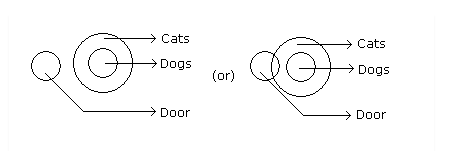# Syllogism

Being a part of the CAT logical reasoning section, the syllogism topic holds a good weightage in the respective section. Almost 3-4 questions are asked every year from syllogism for CAT topic and thus, it should be properly prepared and a wide variety of questions must be practised. Please refer BYJU’S CAT College Predictor tool.

The CAT syllogism questions constitute a set of sentences and conclusions. The candidates are required to understand the sentences and deduce a conclusion from the assumed set of propositions. So, a syllogism can simply be defined as a typical argument that must be arrived at a conclusion through deductive reasoning.

There are two methodologies, Venn Diagrams and analytical method to solve the syllogism questions. The Venn diagram method is preferred since finding the conclusions which follow the given statements logically become easy and thus solving the related syllogism question becomes easy. Diagrams are drawn on the basis of the sentences and then conclusions are drawn by analysing the Venn diagram. An example of solving syllogism questions using Venn diagrams is illustrated for easy understanding of the syllogism questions and the application of the Venn diagram in them.

For example,

Question Statements:

(A) No door is dog.

(B) All the dogs are cats.

Conclusions:

(A) No door is cat.

(B) No cat is door.

(C) Some cats are dogs.

(D) All the cats are dogs.

1. Only (B) and (D)
2. Only (A) and (C)
3. Only (C) and (D)
4. Only (C)
5. All the four

Solution:

From the given question statements, two possible Venn diagrams can be made as,So, answer option 4 i.e. only (C) is the correct option.

#### CAT Syllogism Solutions

Generally, the conclusion statements include 5 important keywords:

• All A are B
• A = B
• No A are B
• Some A are B
• Some A are not B

These statements can be easily understood through Venn diagrams. Visit the link to understand the Venn diagrams properly and solve the syllogism questions with ease.

As the CAT exam includes several syllogism questions every year, it is very crucial to be very well acquainted with the topic and understand to draw Venn diagrams for each statement and draw conclusions from the respective diagrams. If the diagrams are drawn sequentially, deducing the conclusion becomes easy and the questions can be attempted effectively.

To get more such lessons on CAT quantitative aptitude topics, stay tuned with BYJU’S. BYJU’S also provide the latest CAT exam notifications syllabus and pattern along with various tips and strategies to help the CAT aspirants ace the exam with ease.